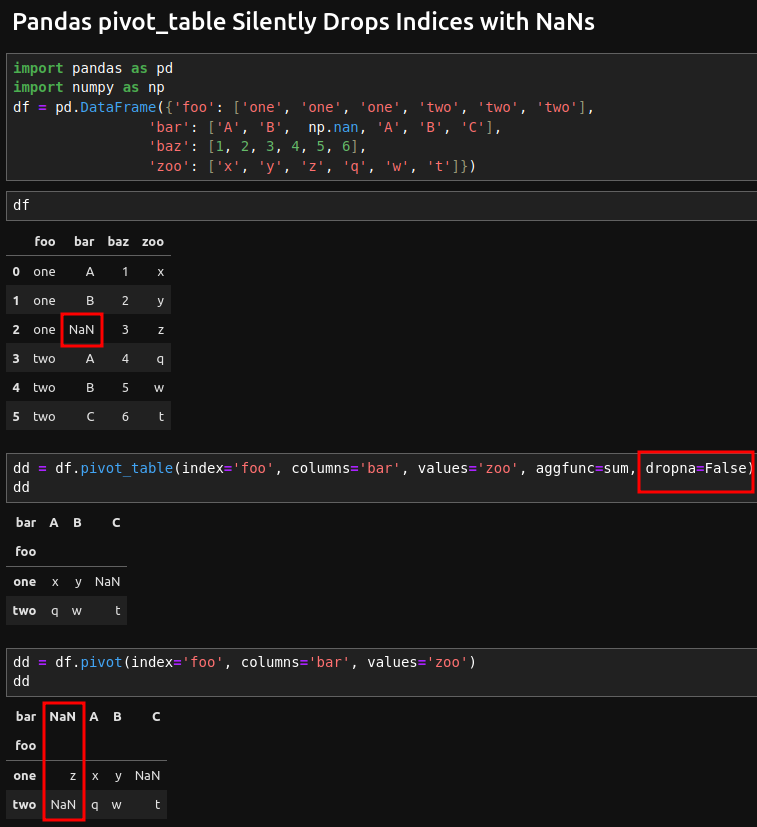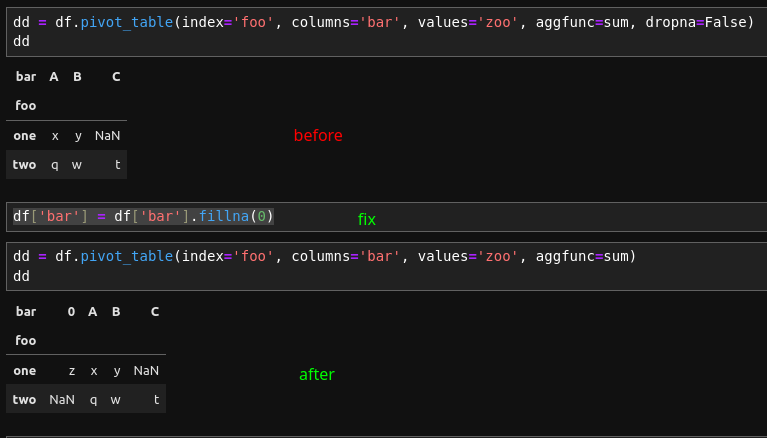In this post, we will discuss when pivot_table silently drops indices with NaN-s. We will give an example, expected behavior and many resources.

## Example

Let's have a DataFrame like:

``````import pandas as pd
import numpy as np
df = pd.DataFrame({'foo': ['one', 'one', 'one', 'two', 'two', 'two'],
'bar': ['A', 'B',  np.nan, 'A', 'B', 'C'],
'baz': [1, 2, 3, 4, 5, 6],
'zoo': ['x', 'y', 'z', 'q', 'w', 't']})
``````

with data:

foo bar baz zoo
0 one A 1 x
1 one B 2 y
2 one NaN 3 z
3 two A 4 q
4 two B 5 w

## silent drop of NaN-s indexes

Now let's run two different examples:

### pivot_table

``````df.pivot_table(index='foo', columns='bar', values='zoo', aggfunc=sum)
``````

result is:

bar A B C
foo
one x y NaN
two q w t

Even trying with `dropna=False` still results in the same behavior in pandas 2.0.1:

``````df.pivot_table(index='foo', columns='bar', values='zoo', aggfunc=sum, dropna=False)
``````

### pivot_table and dropna

Below you can read what is doing parameter `dropna`:

dropna bool, default True

Do not include columns whose entries are all NaN. If True, rows with a NaN value in any column will be omitted before computing margins.

### pivot

while pivot will give us different result:

``````df.pivot(index='foo', columns='bar', values='zoo')
``````

which returns NaN-s from the bar column:

bar nan A B C
foo
one z x y NaN
two NaN q w t## Stop silent drop

Once you analyze the error and data a potential solution might be to fill NaN values with default value (which differs from the rest):

``````df['bar'] = df['bar'].fillna(0)
df.pivot_table(index='foo', columns='bar', values='zoo', aggfunc=sum)
``````

After this change `pivot_table` will not drop the NaN indexes:

foo bar baz zoo
0 one A 1 x
1 one B 2 y
2 one 0 3 z
3 two A 4 q
4 two B 5 w

The image below show the behaviour before and after the silent drop of NaN-s:## Conclusion

You can always refer to the official Pandas documentation for examples and what is expected: Reshaping and pivot tables

Pandas offers a variety of methods and functions to wrangle data. Sometimes the results might be unexpected. In this case test the results against another method or sequence of steps.

If you notice a Pandas bug or unexpected behavior you can open ticket or check Pandas issues like: ENH: pivot/groupby index with nan #3729